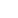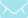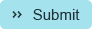Tuesday 10 December 2019
Call sales847-454-3039WhitePapers
WhitePaper - Reorder Point

Reorder Point re-work under various if/then considerations.

The Basics

The primary use of a Reorder Point, or a Minimum / Maximum “range”, is so that under the following conditions, an item will appear on a “Suggested Order”.  The conditions are:

If the Quantity on Hand (QOH) plus the Quantity on Current Purchase Order(s) (COQ) less the Committed to Customers (CCQ) is LESS THAN the Minimum OR Reorder Point, then the system will “suggest” a reorder of the item (SOQ).  The Quantity which is suggested to be reordered is an amount which will take the Quantity on Hand back to the Reorder Point or Maximum Stock Level (MSL), and then rounded by either the Order Multiple (OM), Standard Pack (SP) or whatever amount is defined for an alternative vendor (IMU Screen V).  Mathematically, the formula is:

IF (QOH+COQ-CCQ)< MIN_OP THEN SOQ = MIN_OP -(QOH+COQ-CCQ) * OM (or SP) UNTIL (if applicable) MSL.

When data is taken from the Activant related to inventory records, reorder point calculations can be performed which take into consideration cases that the Activant cannot provide.

Starting with a basic consideration of 4 weeks of supply, and a minimum order point of two (2), then exceptions are individually identified. This minimum order point calculation can also be expressed as:

The Average Number of Units Sold Per Transaction (which is never less than 1) plus the Order Multiple of an item (which is also never less than 1) = 2.

The purpose is to assure that there is always “one to show and one to go”.

The Average Number of Units Sold Per Transaction is an externally calculated amount.  It is computed by taking the Year To Date Number of Transactions (a field available in the Activant) divided by the Year to Date Number of Units Sold (a field also available in the Activant).

The exceptions are listed below.  Additional exceptions can be made, up to and including the establishment of a Minimum / Maximum (Min/Max) which, when the minimum is reached (as per the formula above), the system reorders in sufficient quantity, rounding by either the order multiple or standard pack as required to the maximum level.

Reorder Point Calculation Exceptions:

The first exceptions are those which are defined by the user, and are unique in each situation.  When, for example, there are space limitations, the definition of a Min / Max for the item(s) and PROTECTING (stop the recalculation) the Min/Max is appropriate.

This may be appropriate for VERY high turnover items that can be reordered at will (daily, if necessary).  These items are generally coded as well to provide Stock Alert reports on a daily basis to insure adequate supply.

Another exception is the case of items like expensive power tools, where it may be appropriate to have a reorder point of two (as opposed to one for expensive items) because one is needed for DISPLAY purposes, and one to sell.

Both cases fall outside of calculations based on if/then considerations, and they must be set manually.

Exception A (CALCA): If the cost of an item is greater than \$50, and the Order Multiple is equal to one (1), and the Calculated Order Point at 4 weeks of supply (CALCOP) is less than or equal 1, then the Order Point is equal to one (1).

IF (COST > 50) AND (OM = 1) AND (CALCOP) <= 1 AND (DISCONTINUED) <> “Y”, THEN  (CALCA) = 1

The purpose of this is to minimize stock on expensive, non-display items that 4 weeks of supply is less than or equal 1.

Exception B (CALCB): If the Retail Price of an item is less than \$5.00, and it is an “A” popularity ranking item (it contributes to the first 80% of total sales dollars for a department) and the Order Multiple is one (1) and the Standard Pack is not equal to one (1), then the Reorder Point is equal to the Standard Pack +1.

IF (RETAIL < 5.00) AND (POP = “A;B”) AND (OM=1) AND (STDPK<>1) THEN (CALCB)=(STDPK+1)

The purpose of this is to take advantage of full case pricing, if applicable, and to recognize that restocking / reordering ones or twos of these items is inefficient.  These are generally basic basic items.

Exception C (CALCC): If the company is a lumber yard, then for YARD items with a popularity code of A or B, the minimum reorder point (or minimum in a Min / Max) should be the Standard Pack (unit size for dimensional lumber, plywood, etc) plus one (1).

IF (POP=A;B;C) AND (COMPANYTYPE=L) THEN (CALCC)=(STDPK+1)

The purpose of this is to have a minimum of one ordering unit available at all times.

Exception D (CALCD): If the Average Units per Sale is greater than the basic calculated reorder point, then the reorder point should equal the Average units per sale times two (2).

IF (AVGUNITSALE)>(CALCOP) THEN (CALCD)=(AVGUNITSALE)*2

The purpose of this is to recognize that the average number of units sold when an item is sold is greater than the calculated reorder point.

For example, if the basic calculated reorder point of an item is 2.   But, when an item is sold, a customer purchases an average of 2 of the item.   So, to prevent being completely out of the item, the reorder point would need to be at least four (4).  This way, when the quantity available falls to three, and if a customer comes in to make a purchase, the item is not (theoretically) completely sold out.  The customer could still purchase the average amount sold.

Exception E (CALCE): If the Popularity for an item is C or D, then the reorder point should be the Average Weekly Units Sold times eight (8).

IF (POP=C;D) THEN (CALCE)=(AVGUNITSWK)*8

The purpose of this is a currently prevalent theory that supposes that the reason items become slow sellers is that there is inadequate supply of them.  The customer, seeing only two of an item on the shelf, passes it by as unsuitable, or in an insufficient quantity.  As a result, the “self-fulfilling prophecy” of an item staying a C or D Popularity (or becoming an “X” item) is greatly increased.  The investment is generally minimal, and reinforces the old retailing maxim “You can’t do business from an empty cart”.